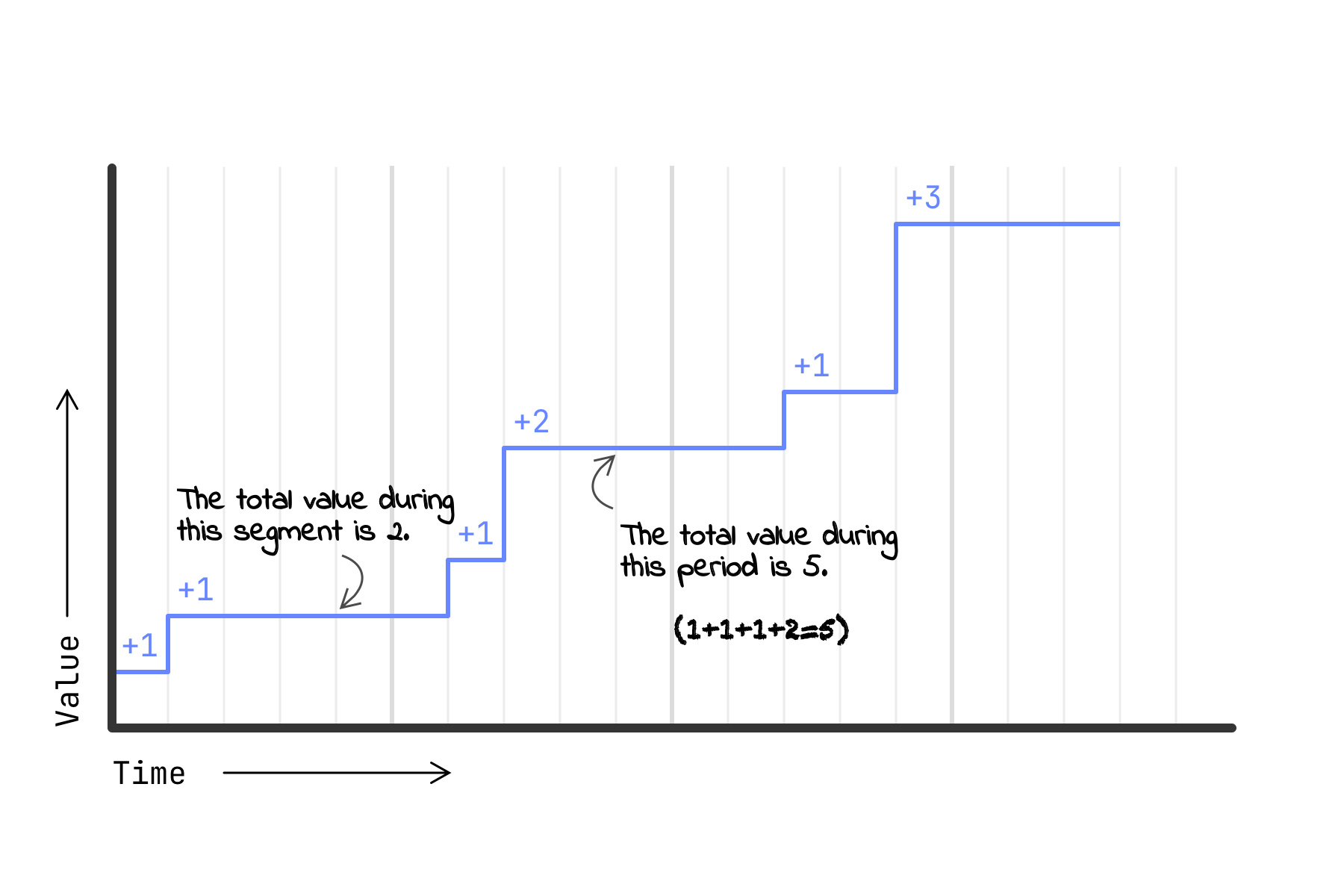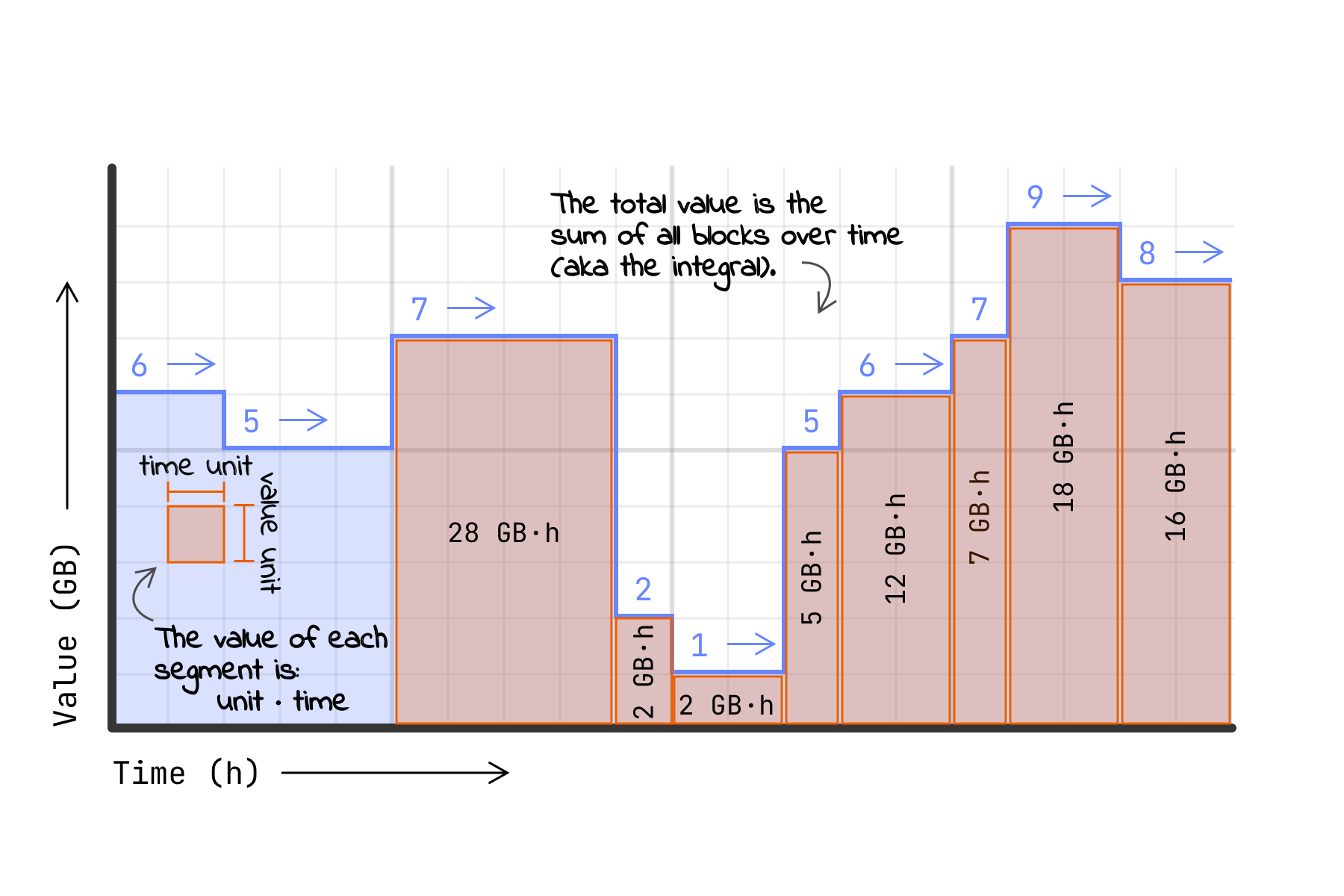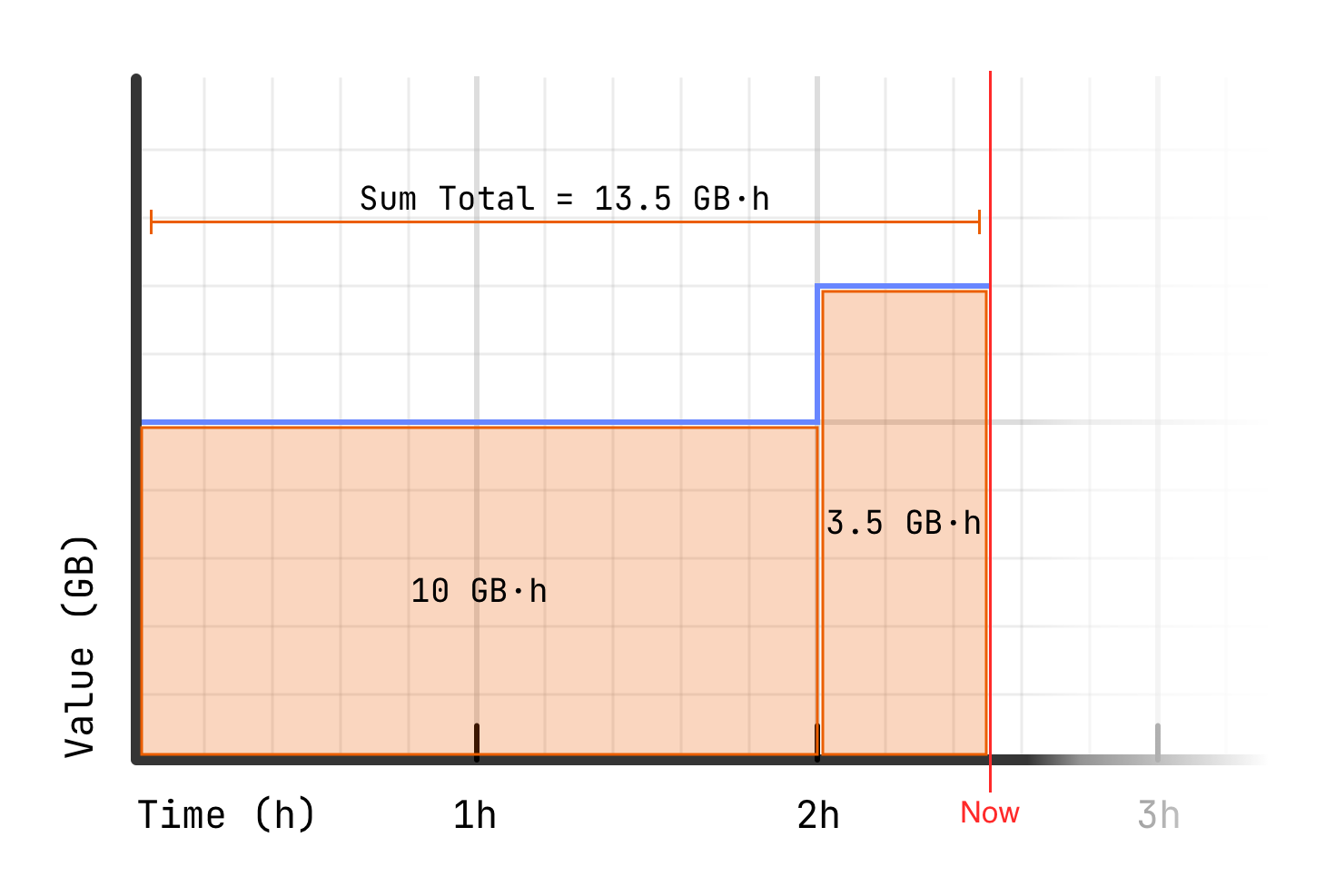# Meter Types

Handling a variety of measurements.

# Why types?

Octane provides two meter types: counters and gauges. The distinction between these is primarily in how they record values in relation to time:

Counter
• Records the number of occurrences of a defined event over a period of time.
• The total value is the sum of all recorded values.
• The unit of the total value is the same as the unit of each measurement.
Gauge
• Records a measured value at many instants over a period of time.
• The total value is the sum of each recorded measurement multiplied by the effective duration of each measurement (the duration between each measurement and the measurement that follows it).
• The unit of the total value is the unit of each measurement multiplied by a unit of time (e.g. `GB·h`).

Analogously, a car's odometer is a counter that tracks the total number of miles travelled, while its speedometer is a gauge displaying the speed at the current instant.

### Working with meters

It's important to note that when you query a customer's usage of a specific meter, the value returned is always the latest recorded value within the queried time period.

``````# Example query for a customer's usage of a specific meter
curl --request GET \
--url 'https://api.cloud.getoctane.io/customers/CUSTOMER/usage?meter_name=METER&end_time=1641672000&start_time=1641668400' \
--header 'Accept: application/json' \
--header 'Authorization: Bearer abcdef123456'
``````

In the case of a counter, the returned value is always the sum of all recorded usage within the queried time period (or within the current billing period if start and end times are not specified).

Things are different for gauges. Rather than an aggregate of all recorded usage within a time period, this endpoint will return the latest value recorded by this meter for this customer, irrespective of how the value may have changed over time.

# Counters

Because a counter's total is the sum of all recorded values, it increases monotonically. This means that the value can only go up over time.This type of meter is used to count the number of occurrences of a given event within a billing period. Counters are typically used to count things like API requests, file uploads, or function invocations.

# Gauges

The value of a gauge can increase or decrease as the measured value recorded by that gauge changes over time. Each value recorded for a given customer on a given gauge is the effective value until another measurement is recorded.This type of meter is used to record measured values as they change over time within a billing period. This adds a time dimension to the meter, which is reflected in the unit used for the aggregate total of a billing period.

Gauges are typically used to record things like the number of active users, the amount of storage provisioned, or the number of running jobs that a customer has, as measured over time. Configuring this type of meter allows Octane to take the burden off of the streaming source to maintain awareness of the time elapsed between measurements.

### Aggregation example

This example demonstrates how total usage is calculated by a gauge:

• The gauge records a value of `5 GB` and that value remains unchanged for two hours. The aggregate usage during that time period is `10 GB·h`.
• Next, the gauge records a value of `7 GB` and remains unchanged for 30 minutes. The usage for that block is `3.5 GB·h` (`7 GB` × `0.5 h`).
• The total usage for that 2.5 hour period is thus `12.5 GB·h`.To put it another way, the total usage for that 2.5 hour period is:

``````(5.0 GB × 2.0 h) +
(3.5 GB × 0.5 h)

= 13.5 GB·h
``````